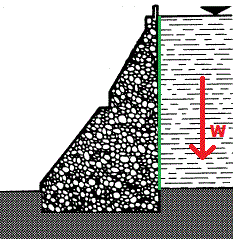# Doubts about the formula for hydrostatic water pressure on a vertical surface

Hey guys,

So I have just learned that the formula for a fluid's hydrostatic pressure (let's say water for the purpose of simplification) in terms of depth is:

P = ρ * g * h

Now I have been reading a bit on my textbook and I found out that the derivation of this formula is based on the assumption that for a given water column with volume V and height h resting on an area A on a given surface, the pressure is:

P = F/A = mg/A = (ρV)g/A = ρ(Ah)g/A = ρgh

Now I've also noticed that the same formula is used even when dealing with vertical contact surfaces, which I don't understand because when in that case (let's say in the case of a dam with a horizontal interface), there is no water directly "resting" on the surface and thus we cannot use the given formula.

So could you please tell me what I have missed in my reasoning and the misunderstandings (if any) that I have about the concept ? Thanks in advance.

(Please note that I am not denying the existence of hydrostatic pressure, I am just saying that its expression shouldn't be that of the conventional ρgh)

SteamKing
Staff Emeritus
Homework Helper
You state:
Now I've also noticed that the same formula is used even when dealing with vertical contact surfaces, which I don't understand because when in that case (let's say in the case of a dam with a horizontal interface), there is no water directly "resting" on the surface and thus we cannot use the given formula.

I'm having trouble making sense of your statement above.

If the dam is not holding the water in the reservoir behind the dam, what is keeping the water contained?

Gold Member
In the case of hydrostatic pressure, the pressure must equal the weight of all fluid pushing down on a given point to satisfy Newton's laws. So basically, you have a column of fluid from the surface to any arbitrary depth, $h$. Let's say that this fluid column has horizontal dimensions $dx_1$ and $dx_2$. The weight of this column is therefore the density times the volume of the column, or $\rho g h \;dx_1 \;dx_2$. This is a force, and we are looking for a pressure, so we just divide it by the area of the base of the column $dx_1 \;dx_2$. That leaves you with:

$$P = \rho g h$$

Since $dx_i$ are infinitesimal, the pressure from that equation represents the pressure at any point in the fluid of depth $h$.

Pressure is actually the force felt as a result of many fluid particles colliding with a surface, and therefore it acts in all directions equally. With that in mind, a vertical surface and a horizontal surface will feel the same force for a given depth, $h$.

SteamKing,

Sorry my bad. When I said
dam with a horizontal interface
, I actually meant "with a vertical interface". Here's an image of the kind of dam I am talking about. I thought that since the weight of the water (in red) is parrallel to the water-dam contact surface (in green), then the water has no effect on it and thus we cannot derive the aforementioned pressure formula (ρgh)You said that:

In the case of hydrostatic pressure, the pressure must equal the weight of all fluid pushing down on a given point to satisfy Newton's laws.

Well I think that in this case, there is no fluid weight pushing down on any point of the surface, correct me if I am wrong ? Thus I still don't think that the derivation is valid. In a nutshell, I believe that your derivation holds when having an inclined or horizontal surface, but not in the case of a vertical one.

HallsofIvy
Homework Helper
The point is that water is a fluid- that is, it exerts the same force in all directions, not just downward.

Gold Member
Well I think that in this case, there is no fluid weight pushing down on any point of the surface, correct me if I am wrong ? Thus I still don't think that the derivation is valid. In a nutshell, I believe that your derivation holds when having an inclined or horizontal surface, but not in the case of a vertical one.

The point is that water is a fluid- that is, it exerts the same force in all directions, not just downward.

This.

That is just how you find the pressure at any point. This includes points that butt up against vertical surfaces, and like I mentioned after that, the nature of a fluid means that the pressure acts in all directions, including horizontally onto the surface it butts up against regardless of the fact that there is no fluid "sitting" on it.

Thank you so much guys, I just re-read Pascal's law and confirmed to myself that pression actually acts equally in all directions. Problem solved !!

sophiecentaur